﻿ 基于智能视觉物联网的教室人数跟踪系统 People Tracking System Based on Intelligent Vision Internet of Things for Classroom

Journal of Image and Signal Processing
Vol. 08  No. 02 ( 2019 ), Article ID: 29962 , 8 pages
10.12677/JISP.2019.82013

People Tracking System Based on Intelligent Vision Internet of Things for Classroom

Xiaoqiang Zhang1,2, Xun Wang1, Hang Yang3, Wei Zeng3

1School of Information and Control Engineering, China University of Mining and Technology, Xuzhou Jiangsu

2Xuzhou Key Laboratory of Artificial Intelligence and Big Data, Xuzhou Jiangsu

3School of Electrical and Power Engineering, China University of Mining and Technology, Xuzhou Jiangsu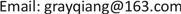Received: Apr. 3rd, 2019; accepted: Apr. 14th, 2019; published: Apr. 28th, 2019ABSTRACT

To count the number of people in the classroom in real time, the classroom video monitoring system is used to generate the real-time images in the classroom. A reliable and stable people tracking application platform is realized in this paper. Taking into account the people distribution characteristics in the classroom and the architectural style of the classroom, this paper adopts a head detecting algorithm. The extracted video frame HOG sample features are then trained using the AdaBoost cascade classifier to implement a head detection model for detecting the number of people. Finally, the mobile client based on the Android platform is developed for the user to query the number of people in the classroom in real time. System testing results show that the system has an excellent recognition rate. In the case of not hiding the head characteristics, the system recognition rate is more than 75%, which can basically satisfy the requirements of people counting in real time.

1中国矿业大学信息与控制工程学院，江苏 徐州

2徐州市人工智能与大数据重点实验室，江苏 徐州

3中国矿业大学电气与动力工程学院，江苏 徐州1. 引言

2. 人数统计技术综述

3. 理论依据

3.1. HOG特征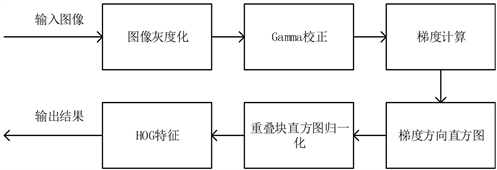Figure 1. The detection process of HOG feature

${C}_{m}\left(x\right)={a}_{1}{k}_{1}\left(x\right)+{a}_{2}{k}_{2}\left(x\right)+\cdots +{a}_{m}{k}_{m}\left(x\right),$ (1)

4. 系统设计与实现

4.1. 图像获取

$I=0.299*R+0.587*G+0.114*B,$ (2)

$Y\left(x,y\right)=I\left(x,y\right),$ (3)

4.2. 人数检测

${G}_{x}\left(x,y\right)=I\left(x+1,y\right)-I\left(x-1,y\right),$ (4)

${G}_{y}\left(x,y\right)=I\left(x,y+1\right)-I\left(x,y-1\right).$ (5)

${m}_{i}\left(x,y\right)=\sqrt{{G}_{x}{\left(x,y\right)}^{2}+{G}_{y}{\left(x,y\right)}^{2}},$ (6)

$\theta \left(x,y\right)=\mathrm{arctan}\left(\frac{{G}_{y}\left(x,y\right)}{{G}_{x}\left(x,y\right)}\right).$ (7)

$M\left(x,y\right)={\sum }_{i=1}^{N}{m}_{i}\left(x,y\right).$ (8)

$M$ 进行归一化处理：

$\stackrel{˜}{M}\left(x,y\right)=\frac{M\left(x,y\right)}{\mathrm{max}\mathrm{max}M\left(x,y\right)}×255.$ (9)

$\beta ←\frac{\beta }{\sqrt{{\beta }^{2}+{\epsilon }^{2}}}.$ (10)

1) 首先初始化样本权重 $W\left(i\right)=1/N$

2) 在默认权重 $W$ 和样本集 $C$ 下，对每个特征 $f\in FC$ ，训练弱分类器 ${k}_{i}\left(x\right)$ ，并选取出最优的弱分类器；

3) 更新权重 $W$

4) 输出强分类器： ${C}_{m}\left(x\right)={a}_{1}{k}_{1}\left(x\right)+{a}_{2}{k}_{2}\left(x\right)+\cdots +{a}_{m}{k}_{m}\left(x\right)$

4.3. 数据推送

5. 系统测试与结果分析

5.1. 系统测试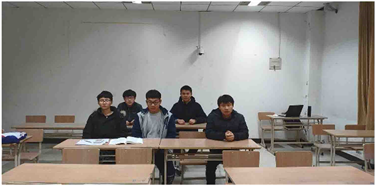Figure 2. Original image of video frame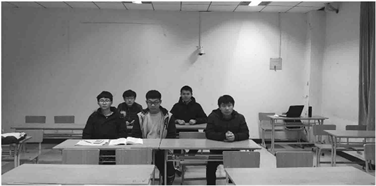Figure 3. Pretreatment result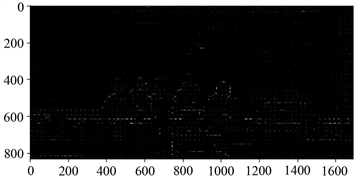Figure 5. Histogram of oriented gradient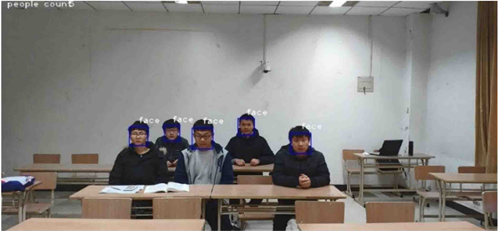Figure 6. System result of test

5.2. 结果分析

5.2.1. 系统识别率分析

$R=\frac{|教室实际人数-系统统计人数|}{教室实际人数}×100%.$ (11)

5.2.2. 系统延时分析

6. 结论与展望

People Tracking System Based on Intelligent Vision Internet of Things for Classroom[J]. 图像与信号处理, 2019, 08(02): 90-97. https://doi.org/10.12677/JISP.2019.82013

1. 1. 苏娟. 基于视频监控的教室人数统计[D]: [硕士学位论文]. 合肥: 安徽大学, 2014.

2. 2. 刘砚. 基于hog特征的教室人数拥挤度检测系统的设计与实现[D]: [硕士学位论文]. 武汉: 华中科技大学, 2015.

3. 3. 黄雯, 张为, 付晓梅, 张泰. 一种基于多分类器融合的人数统计算法[J]. 南开大学学报(自然科学版), 2019, 52(1): 15-20.

4. 4. 郭继昌, 李翔鹏. 基于卷积神经网络和密度分布特征的人数统计方法[J]. 电子科技大学学报, 2018, 47(6): 806-813.

5. 5. Liu, G.Q., Zhong, S.H. and Li, T. (2019) Gait Recognition Method of Temporal-Spatial HOG Features in Critical Separation of Fourier Correction Points. Future Generation Computer Systems, 94, 11-15.
https://doi.org/10.1016/j.future.2018.09.012

6. 6. 张宇洋, 刘满华, 韩韬. 基于Mean Shift图像分割和支持向量机判决的候梯人数视觉检测系统[J]. 光学精密工程, 2013, 21(4): 1079-1085.

7. 7. Dalal, N. and Triggs, B. (2005) Histograms of Oriented Gradients for Human Detection. 2005 IEEE Computer Society Conference on Computer Vision and Pattern Recognition, San Diego, 20-25 June 2005, 886-893.
https://doi.org/10.1109/cvpr.2005.177

8. 8. Cheng, H.S. and Hu, X.F. (2012) The Humanbody Detection Based on HOG and SVM Technology Research in Static Image. Chinese Journal of Scientific Instrument, 19, 20-23.

9. 9. 龙伶敏. 基于Adaboost的人脸检测方法及眼睛定位算法研究[D].: [硕士学位论文]. 成都: 电子科技大学, 2008.

10. 10. 郭磊, 王秋光. Adaboost人脸检测算法研究及OpenCV实现[J]. 哈尔滨理工大学学报, 2009, 14(5): 123-126.

11. 11. 刘威, 段成伟, 遇冰, 柴丽颖, 袁淮, 赵宏. 基于后验HOG特征的多姿态行人检测[J]. 电子学报, 2015, 43(2): 217-224.

12. 12. 黎向锋, 赵伟康, 豆心愿, 等. 基于改进AdaBoost + Haar的车辆检测算法[J]. 测控技术, 2019, 38(2): 42-45.

13. 13. 曾波波, 王贵锦,林行刚. 基于颜色自相似度特征的实时行人检测[J]. 清华大学学报(自然科学版), 2012, 52(4): 571-574.